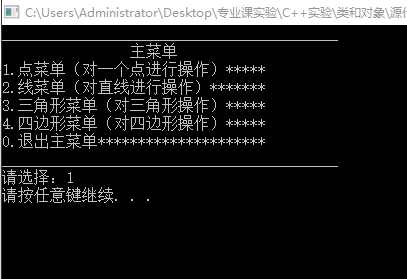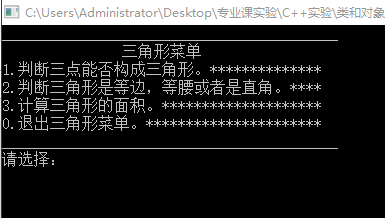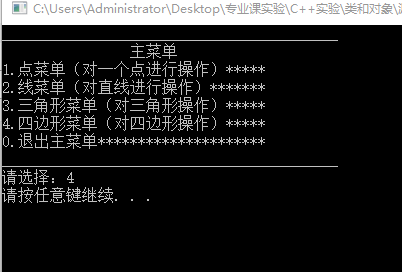• 华东师大版八年级数学下册导学案：18.1 第4课时 平行四边形周长面积的相关计算.docx
• 求任意三角形和四边形面积都可以用行列式来求，其他几边形也都可以，这里就不多写了。（this is my first 博客。目前还很菜） 在这里插入代码片 package 求面积;//(如果父类中有与子类相同的方法，那么子类...

> 求任意三角形和四边形的面积都可以用行列式来求，其他几边形也都可以，这里就不多写了。（this is my first 博客。目前还很菜）

在这里插入代码片
package 求面积;//(如果父类中有与子类相同的方法，那么子类向上转型之后，用对象的引用可以调用子类中的方法。但是如果父类中没有和子类相同的方法，则无法调用子类中的方法。
import java.util.Scanner;
public class Main {
static Scanner cin=new Scanner(System.in);
public static void main(String[] args)
{
while(cin.hasNext()) {
int x=cin.nextInt();
if(x==1) {
Triangle t=new Triangle();
t.p=new Point;//记得对象数组，要对每个对象都实例化。
for(int i=0;i<3;i++) {
t.p[i]=new Point();
t.p[i].x=cin.nextDouble();
t.p[i].y=cin.nextDouble();
}
System.out.printf("这是一个三角形,周长为:%.1f,面积为%.1f.\n",t.perimeter(t),t.area(t));
}
if(x==2) {
Rectangle t=new Rectangle();
t.p=new Point;
mpoint(t);//每个都有，直接写成方法来调用。
System.out.printf("这是一个矩形,周长为:%.1f,面积为%.1f.\n",t.perimeter(t),t.area(t));
}
if(x==3) {
Trapezoid t=new Trapezoid();
t.p=new Point;
mpoint(t);
System.out.printf("这是一个梯形,周长为:%.1f,面积为%.1f.\n",t.perimeter(t),t.area(t));
}
if(x==4) {
Rhomboid t=new Rhomboid();
t.p=new Point;
mpoint(t);
System.out.printf("这是一个平行四边形,周长为:%.1f,面积为%.1f.\n",t.perimeter(t),t.area(t));
}

if(x==5) {
Diamond t=new Diamond();
t.p=new Point;
mpoint(t);
System.out.printf("这是一个菱形,周长为:%.1f,面积为%.1f.\n",t.perimeter(t),t.area(t));
}
}
}
public static void mpoint(Playgon t) {
for(int i=0;i<4;i++) {
t.p[i]=new Point();
t.p[i].x=cin.nextDouble();
t.p[i].y=cin.nextDouble();
}
}
}
class Point{
double x,y;
public Point() {
super();
}
public double distance(Point p1,Point p2) {
double distance;
distance =Math.sqrt( Math.pow((p1.x-p2.x),2)+ Math.pow((p1.y-p2.y),2));
return distance;
}
}
class Playgon{
Point p[];
public Playgon() {

}
}
class Triangle extends Playgon{
public Triangle() {
super();
}
public double perimeter(Triangle t) {
double l=0;
l=t.p.distance(t.p, t.p)+new Point().distance(t.p, t.p)+new Point().distance(t.p,t.p);
return l;
}
public double area(Triangle t) {
double s=0;
for(int i=0;i<3;i++) {
if(i==2) {
s=s+t.p[i].x*t.p.y-t.p.x*t.p[i].y;
}
else {
s=s+t.p[i].x*t.p[i+1].y-t.p[i+1].x*t.p[i].y;
}
}
return s/2;//行列式啊求三角形面积。
}
}
class Rectangle extends Playgon{
public Rectangle() {
super();
}
public double perimeter(Rectangle t) {
double l=0;
l=new Point().distance(t.p, t.p)+new Point().distance(t.p, t.p)+new Point().distance(t.p, t.p)+new Point().distance(t.p, t.p);//四边形周长，四个边相加，通用。
return l;
}
public double area(Rectangle t) {
double s=0;
for(int i=0;i<4;i++) {
if(i==3) {
s=s+t.p[i].x*t.p.y-t.p.x*t.p[i].y;
}
else {
s=s+t.p[i].x*t.p[i+1].y-t.p[i+1].x*t.p[i].y;
}
}
return s/2;//行列式求四边形面积。（通用）。
}
}
class Trapezoid extends Rectangle{
public Trapezoid() {
super();
}
}
class Rhomboid extends Trapezoid{
public Rhomboid() {
super();
}
}
class Diamond extends Rhomboid{//由于类中的方法一样，可以连续继承。
public Diamond() {
super();
}
}



展开全文多态 面向对象编程
• 给定一个平行四边形的底边长度 l 高度 h，求平行四边形面积。 【输入格式】 输入的第一行包含一个整数 l，表示平行四边形的底边长度。 第二行包含一个整数 h，表示平行四边形的高。 【输出格式】 输出一个整数，...
第十二届蓝桥杯第二次模拟赛
试题 F
时间限制: 1.0s 内存限制: 512.0MB 本题总分：15 分
【问题描述】
给定一个平行四边形的底边长度 l 和高度 h，求平行四边形的面积。
【输入格式】
输入的第一行包含一个整数 l，表示平行四边形的底边长度。
第二行包含一个整数 h，表示平行四边形的高。
【输出格式】
输出一个整数，表示平行四边形的面积。（提示：底边长度和高都是整数的平行四边形面积为整数）
【样例输入】
2
7
【样例输出】
14
【评测用例规模与约定】
对于所有评测用例，1 <= l, h <= 100。
package _12十二届模拟赛02;

import java.util.Scanner;

public class Test06求平行四边形的面积 {
public static void main(String[] args) {
Scanner sc = new Scanner(System.in);
System.out.println(sc.nextInt()*sc.nextInt());
}
}




展开全文• 1.类对象简介 类对象的关系：类是对象的抽象，而对象是类的特例，即类的具体表现形式。 类对象的区别：类是对象的模板，类是抽象的不占用内存，对象是具体的，占用储存空间。 类中主要包括数据成员函数...
1.类和对象简介
类和对象的关系：类是对象的抽象，而对象是类的特例，即类的具体表现形式。
类和对象的区别：类是对象的模板，类是抽象的不占用内存，对象是具体的，占用储存空间。
类中主要包括数据和成员函数（通常情况下数据设置为私有，成员函数通常设为共用，因为成员函数通常作为外界的接口）
注意：声明类一定要放在对象之前
2.程序实例
1.程序简介
下面是一个实例
程序功能：
利用点、线、面的基本知识，声明Point,Line,Friangle,PolyAngle四个类，完成以下功能。
Point类功能：
（1） 移动一个点；
（2） 显示一个点；
（3） 可计算这个点到原点的距离。
Line类功能：
（1） 计算点到直线的距离；
（2） 直线的斜率；
（3） 判断两条直线的关系(平行/相交（交点坐标能够给出来并显示）/垂直)；
Friangle类功能：
（1） 判断三点能否构成三角形；
（2） 判断三角形是等边，等腰或者是直角；
（3） 计算三角形的面积。
PolyAngle类功能：
（1） 判断四点能否构成四边形，并判断其是凹的，还是凸的；
（2） 判断四边形是否是等腰梯形；
（3） 判断四边形是否是平行四边形，菱形；
（4） 判断它是否是一个矩形；
（5） 计算四边形的面积和周长。
2. 源代码
在这里插入代码片
#include<iostream>
#include<cmath>// 数学计算要用到此头文件
#include <cstdlib>//暂停和清屏函数要用到此头文件
using namespace std;
class Point//点类
{
public:
Point()//构造函数
{
x=0;
y=0;
}
void f1();//输入A点的坐标函数
void f2();//输入A点移动量的函数
void f3();//输出点A的坐标函数
void f4();//计算A点到原点的距离
private:
double x;
double y;
};
void Point::f1()//输入A点的坐标函数
{
cout<<"请输入A点的坐标（两个数中间用空格隔开）：";
cin>>x>>y;
}
void Point::f2()//输入A点移动量的函数
{
double x1,y1;//x1和y1表示 A点在x轴和y轴上的增量
cout<<"请依次输入A点在x轴和y轴上的增量（两个数中间用空格隔开）：";
cin>>x1>>y1;
x=x+x1;
y=y+y1;
}
void Point::f3()//输出A点此时的坐标函数
{
cout<<"此时A点的坐标为："<<"("<<x<<","<<y<<")"<<endl;
}
void Point::f4()//计算A点到原点的距离
{
double d;
d=sqrt((x-0)*(x-0)+(y-0)*(y-0));//计算A点到原点的距离
cout<<"此时A点到原点的距离为:"<<d<<endl;
}
class Line//线类
{
public:
Line()//构造函数
{
ax=bx=cx=dx=ex=0;
ay=by=cy=dy=ey=0;
}
void f1();// 计算点到直线的距离
void f2();// 计算直线的斜率
void f3();// 计算两条直线的关系
private:
double ax,bx,cx,dx,ex;
double ay,by,cy,dy,ey;
/*定义5个点a点是动点，bc点构成第一条直线，de点构成第二条直线*/
};
void Line::f1()// 计算点到直线的距离
{
double d,d1,d2,k,b;
cout<<"请输入a点的坐标（两个数中间用空格隔开）：";
cin>>ax>>ay;
cout<<"开始输入直线（请按照提示操作）"<<endl;
cout<<"请输入直线上的2个不同的点(例如:1 2 2 0)：";
cin>>bx>>by>>cx>>cy;
while(bx==cx&&by==cy)//判断输入的两个点是否相同
{
cout<<"输入的2个点相同!"<<endl<<"请重新输入:";
cin>>bx>>by>>cx>>cy;
}
cout<<"输入的b，c两点坐标分别为:"<<"b("<<bx<<","<<by<<"),"<<"c("<<cx<<","<<cy<<")"<<endl;
if(bx==cx)//直线斜率不存在
{
d=ax-bx;//点a到直线的距离
if(d<0)
{
d=-d;
}
if(bx>=0)
{
cout<<"直线的方程为：x-"<<bx<<"=0";
cout<<"点a"<<"("<<ax<<","<<ay<<")"<<"到直线x-"<<bx<<"=0"<<"的距离为d,d="<<d<<endl;
}
else
{
cout<<"直线的方程为：x+"<<-bx<<"=0";
cout<<"点a"<<"("<<ax<<","<<ay<<")"<<"到直线x+"<<-bx<<"=0"<<"的距离为d,d="<<d<<endl;
}
}
else//斜率存在
{
k=(by-cy)/(bx-cx);
b=cy-k*cx;
d1=k*ax+(-1)*ay+b;
d2=sqrt(k*k+-1*-1);
if(d1<0)
{
d=-d1/d2;
}
else
{
d=d1/d2;
}
if(b>=0)
{
cout<<"直线的方程为:"<<k<<"x-y+"<<b<<"=0"<<endl;
cout<<"点a"<<"("<<ax<<","<<ay<<")"<<"到直线"<<k<<"x-y+"<<b<<"=0的距离为d,d="<<d<<endl;
}
else
{
cout<<"直线的方程为:"<<k<<"x-y"<<b<<"=0"<<endl;
cout<<"点a"<<"("<<ax<<","<<ay<<")"<<"到直线"<<k<<"x-y"<<b<<"=0的距离为d,d="<<d<<endl;
}

}
}
void Line::f2()// 计算直线的斜率
{
double k,b;
cout<<"开始输入直线的方程（请按照提示操作）"<<endl;
cout<<"请输入直线上的2个不同的点(例如:1 2 2 0)：";
cin>>bx>>by>>cx>>cy;
while(bx==cx&&by==cy)//判断输入的两个点是否相同
{
cout<<"输入的2个点相同!"<<endl<<"请重新输入:";
cin>>bx>>by>>cx>>cy;
}
cout<<"输入的b，c两点坐标分别为:"<<"b("<<bx<<","<<by<<"),"<<"c("<<cx<<","<<cy<<")"<<endl;
if(bx==cx)//直线斜率不存在
{
if(bx>=0)
{
cout<<"直线的方程为：x-"<<bx<<"=0";
cout<<"直线x-"<<bx<<"=0"<<"的斜率不存在"<<endl;
}
else
{
cout<<"直线的方程为：x+"<<-bx<<"=0";
cout<<"直线x+"<<-bx<<"=0"<<"的斜率不存在"<<endl;
}
}
else//斜率存在
{
k=(by-cy)/(bx-cx);
b=cy-k*cx;
if(b>=0)
{
cout<<"直线的方程为:"<<k<<"x-y+"<<b<<"=0"<<endl;
cout<<"直线"<<k<<"x-y+"<<b<<"=0的斜率为k,k="<<k<<endl;
}
else
{
cout<<"直线的方程为:"<<k<<"x-y"<<b<<"=0"<<endl;
cout<<"直线"<<k<<"x-y"<<b<<"=0的斜率为k,k="<<k<<endl;
}
}
}
void Line::f3()// 计算两条直线的关系
{
double k,k1,b,b1,x0,y0;
cout<<"开始输入第1条直线的方程（请按照提示操作）"<<endl;
cout<<"请输入直线上的2个不同的点(例如:1 2 2 0)：";
cin>>bx>>by>>cx>>cy;
while(bx==cx&&by==cy)//判断输入的两个点是否相同
{
cout<<"输入的2个点相同!"<<endl<<"请重新输入:";
cin>>bx>>by>>cx>>cy;
}
cout<<"输入的b，c两点坐标分别为:"<<"b("<<bx<<","<<by<<"),"<<"c("<<cx<<","<<cy<<")"<<endl;
if(bx==cx)//直线斜率不存在
{
if(bx>=0)
{
cout<<"第1条直线的方程为：x-"<<bx<<"=0"<<endl;
}
else
{
cout<<"第1条直线的方程为：x+"<<-bx<<"=0"<<endl;
}
}
else//斜率存在
{
k=(by-cy)/(bx-cx);
b=cy-k*cx;
if(b>=0)
{
cout<<"第1条直线的方程为:"<<k<<"x-y+"<<b<<"=0"<<endl;
}
else
{
cout<<"第1条直线的方程为:"<<k<<"x-y"<<b<<"=0"<<endl;
}
}
cout<<"开始输入第2条直线的方程（请按照提示操作）"<<endl;
cout<<"请输入直线上的2个不同的点(例如:1 2 2 0)：";
cin>>dx>>dy>>ex>>ey;
while(dx==ex&&dy==ey)//判断输入的两个点是否相同
{
cout<<"输入的2个点相同!"<<endl<<"请重新输入:";
cin>>dx>>dy>>ex>>ey;
}
cout<<"输入的d，e两点坐标分别为:"<<"d("<<dx<<","<<dy<<"),"<<"e("<<ex<<","<<ey<<")"<<endl;
if(dx==ex)//直线斜率不存在
{
if(dx>=0)
{
cout<<"第2条直线的方程为：x-"<<dx<<"=0"<<endl;
}
else
{
cout<<"第2条直线的方程为：x+"<<-dx<<"=0"<<endl;
}
}
else//斜率存在
{
k1=(dy-ey)/(dx-ex);
b1=dy-k1*dx;
if(b1>=0)
{
cout<<"第2条直线的方程为:"<<k1<<"x-y+"<<b1<<"=0"<<endl;
}
else
{
cout<<"第2条直线的方程为:"<<k1<<"x-y"<<b1<<"=0"<<endl;
}
}/*以上代码是输入两条直线*/
system ("pause");//暂停
system("cls");//清屏
/*以下代码是输出两条直线*/
if(bx==cx)//直线斜率不存在
{
if(bx>=0)
{
cout<<"第1条直线的方程为：x-"<<bx<<"=0"<<endl;
}
else
{
cout<<"第1条直线的方程为：x+"<<-bx<<"=0"<<endl;
}
}
else//斜率存在
{
if(b>=0)
{
cout<<"第1条直线的方程为:"<<k<<"x-y+"<<b<<"=0"<<endl;
}
else
{
cout<<"第1条直线的方程为:"<<k<<"x-y"<<b<<"=0"<<endl;
}
}
if(dx==ex)//直线斜率不存在
{
if(dx>=0)
{
cout<<"第2条直线的方程为：x-"<<dx<<"=0"<<endl;
}
else
{
cout<<"第2条直线的方程为：x+"<<-dx<<"=0"<<endl;
}
}
else//斜率存在
{
if(b1>=0)
{
cout<<"第2条直线的方程为:"<<k1<<"x-y+"<<b1<<"=0"<<endl;
}
else
{
cout<<"第2条直线的方程为:"<<k1<<"x-y"<<b1<<"=0"<<endl;
}
}
/*以下代码是计算两条直线的位置关系*/
if(bx==cx)//第一条直线斜率不存在
{
if(dx==ex)//第二条直线斜率不存在
{
if(bx==dx)
{
cout<<"这两条直线平行且重合。"<<endl;
}
else
{
cout<<"这两条直线平行。"<<endl;
}
}
else//第二条直线斜率存在
{
if(k1==0)
{
x0=bx;
y0=dy;
cout<<"这两条直线相交且垂直。"<<endl;
cout<<"交点坐标为："<<"("<<x0<<","<<y0<<")"<<endl;
}
else
{
x0=bx;
y0=k1*x0+b1;
cout<<"这两条直线相交。"<<endl;
cout<<"交点坐标为："<<"("<<x0<<","<<y0<<")"<<endl;
}
}
}
else//第一条直线斜率存在
{
if(dx==ex)//第二条直线斜率不存在
{
if(k==0)
{
x0=dx;
y0=by;
cout<<"这两条直线相交且垂直。"<<endl;
cout<<"交点坐标为："<<"("<<x0<<","<<y0<<")"<<endl;
}
else
{
x0=dx;
y0=k*x0+b;
cout<<"这两条直线相交。"<<endl;
cout<<"交点坐标为："<<"("<<x0<<","<<y0<<")"<<endl;
}
}
else//第二条直线斜率存在
{
if(k==k1)
{
if(b==b1)
{
cout<<"这两条直线平行且重合。"<<endl;
}
else
{
cout<<"这两条直线平行。"<<endl;
}
}
else
{
if((k*k1)==(-1))
{
x0=(b1-b)/(k-k1);
y0=k*x0+b;
cout<<"这两条直线相交且垂直。"<<endl;
cout<<"交点坐标为："<<"("<<x0<<","<<y0<<")"<<endl;
}
else
{
x0=(b1-b)/(k-k1);
y0=k*x0+b;
cout<<"这两条直线相交。"<<endl;
cout<<"交点坐标为："<<"("<<x0<<","<<y0<<")"<<endl;
}
}
}
}
}
class Friangle
{
public:
Friangle()//构造函数
{
ax=bx=cx=0;
ay=by=cy=0;
}
int f1();// 判断三点能否构成三角形。
void f2();// 判断三角形是等边，等腰或者是直角。
void f3();//计算三角形的面积。
void f4();//输入三个点的函数
void f5();// 判断三角形是等边，等腰或者是直角。子函数
void f6();//计算三角形的面积。子函数
private:
double ax,bx,cx;
double ay,by,cy;
double C,C1,Cab,Cac,Cbc;
double s;
};
int Friangle::f1()//判断三点能否构成三角形。
{
int i;
double k,b;
if((ax==bx&&ay==by)||(ax==cx&&ay==cy)||(bx==cx&&by==cy))
{
cout<<"3个点之中至少有2个点重合，故这三个点不能构成三角形"<<endl;
}
else
{
if(bx==cx)//直线斜率不存在
{
//此时bc直线方程为x=bx;
if(ax==bx)
{
cout<<"abc在一条直线上，故无法构成三角形！"<<endl;
i=0;
}
else
{
cout<<"abc三点能够成三角形。"<<endl;
i=1;
}
}
else//斜率存在
{
k=(by-cy)/(bx-cx);
b=cy-k*cx;
if(ay==(ax*k+b))
{
cout<<"abc在一条直线上，故无法构成三角形！"<<endl;
i=0;
}
else
{
cout<<"abc三点能够成三角形。"<<endl;
i=1;
}
}
}
return i;
}
void Friangle::f2()// 判断三角形是等边，等腰或者是直角。
{
int n,i;
do
{
cout<<"此时abc三个点的坐标分别为"<<"a("<<ax<<","<<ay<<")"<< "b("<<bx<<","<<by<<")"<<"c("<<cx<<","<<cy<<")"<<endl;
cout<<"1.判断当前三个点能否构成三角形，再判断三角形的形状"<<endl;
cout<<"2.重新输入三个点判断能否构成三角形，再判断三角形的形状" <<endl;
cout<<"请选择：";
cin>>n;
switch(n)
{
case 1:system ("pause"); system("cls");i=f1();break;
case 2: system ("pause"); system("cls");f4();i=f1();break;
default :cout<<"输入有误！将要重新选择"<<endl;system ("pause");system("cls");
}
}while(n!=1&&n!=2);
if(i==1)
{
f5();
}
}
void Friangle::f3()//计算三角形的面积。
{
int n,i;
do
{
cout<<"此时abc三个点的坐标分别为"<<"a("<<ax<<","<<ay<<")"<< "b("<<bx<<","<<by<<")"<<"c("<<cx<<","<<cy<<")"<<endl;
cout<<"1.判断当前三个点能否构成三角形，再计算三角形的面积"<<endl;
cout<<"2.重新输入三个点判断能否构成三角形，再计算三角形的面积" <<endl;
cout<<"请选择：";
cin>>n;
switch(n)
{
case 1:system ("pause"); system("cls");i=f1();break;
case 2: system ("pause"); system("cls");f4();i=f1();break;
default :cout<<"输入有误！将要重新选择"<<endl;system ("pause");system("cls");
}
}while(n!=1&&n!=2);
if(i==1)
{
f6();
}
}
void Friangle::f4()//输入三个点的函数
{
cout<<"请输入三个点的坐标(例如：1 0 2 0 0 0)：" ;
cin>>ax>>ay>>bx>>by>>cx>>cy;
}
void Friangle::f5()// 判断三角形是等边，等腰或者是直角。子函数
{
double a;
double t;
int i,j;
Cab=sqrt((ax-bx)*(ax-bx)+(ay-by)*(ay-by));
Cac=sqrt((ax-cx)*(ax-cx)+(ay-cy)*(ay-cy));
Cbc=sqrt((bx-cx)*(bx-cx)+(by-cy)*(by-cy));
a=(ax-bx)*(ax-bx)+(ay-by)*(ay-by);//令a=Cab*Cab;
a=(ax-cx)*(ax-cx)+(ay-cy)*(ay-cy);
a=(bx-cx)*(bx-cx)+(by-cy)*(by-cy);
for(i=0;i<2;i++)//冒泡排序对三角形的三个边进行排序
{
for(j=0;j<2-i;j++)
{
if(a[i]>a[i+1])
{
t=a[i];
a[i]=a[i+1];
a[i+1]=t;
}
}
}
cout<<"三角形的三个点的坐标分别为："<<endl;
cout<<"a点坐标：a("<<ax<<","<<ay<<")"<<endl;
cout<<"b点坐标：b("<<bx<<","<<by<<")"<<endl;
cout<<"c点坐标：c ("<<cx<<","<<cy<<")"<<endl;
cout<<"三角形的三个边长及变长的平方分别为："<<endl;
cout<<"ab="<<Cab<<" ac"<<Cac<<" bc"<<Cbc<<endl;
cout<<"ab*ab="<<a<<" ac*ac="<<a<<" bc*bc="<<a<<endl;
if(Cab==Cac||Cab==Cbc||Cbc==Cac)
{
if(Cab==Cac&&Cab==Cbc)
{
cout<<"这个三角形为等腰三角形，并且为等边三角形"<<endl;
}
else
{
if(a==a+a)
{
cout<<"这个三角形为等腰直角三角形"<<endl;
}
else
{
cout<<"这个三角形为等腰三角形，但不是等边三角形"<<endl;
}
}
}
else
{
if(a==a+a)
{
cout<<"这个三角形为普通的直角三角形"<<endl;
}
else
{
cout<<"这个三角形为普通的三角形"<<endl;
}
}
}
void Friangle::f6()//计算三角形的面积。子函数
{
Cab=sqrt((ax-bx)*(ax-bx)+(ay-by)*(ay-by));
Cac=sqrt((ax-cx)*(ax-cx)+(ay-cy)*(ay-cy));
Cbc=sqrt((bx-cx)*(bx-cx)+(by-cy)*(by-cy));
C=Cab+Cac+Cbc;
C1=C/2;
s=sqrt(C1*(C1-Cab)*(C1-Cac)*(C1-Cbc));
cout<<"三角形的面积为：s,s="<<s<<endl;
}
class PolyAngle
{
public:
PolyAngle()//构造函数
{
ax=bx=cx=dx=0;
ay=by=cy=dy=0;
}
void f1();//判断四点能否构成四边形,并判断其是凹的，还是凸的。
void f1a();//输入四个点的函数
void f1b();//调用计算函数
int f1c();// 计算函数
int f1d(double a1x,double a1y,double b1x,double b1y,double c1x,double c1y);// 计算函数2
void f1e();//判断四边形的凹凸
double f1f(double a1x,double a1y,double b1x,double b1y,double c1x,double c1y);//计算小三角形面积
void f2();// 判断四边形的形状 1
void f2a();// 判断四边形的形状 2
void f2b();//判断6组边的关系
int f2c(double x1,double y1,double x2,double y2,double x3,double y3,double x4,double y4);//判断两条直线的关系
void f2d();//判断四边形的形状 3
void f3();//  计算四边形的面积和周长
private:
double ax,bx,cx,dx;
double ay,by,cy,dy;
double C,Cacb,Cacd,Cabd,Ccbd,Cacb1,Cacd1,Cabd1,Ccbd1,Cab,Cbc,Ccd,Cda,Cac,Cbd;
double s,sacb,sacd,sabd,scbd,sacb1,sacd1,sabd1,scbd1;
int q;//q=0表示四个点无法构成四边形q=1表示四个点能构成四边形
};
void PolyAngle::f1()//判断四点能否构成四边形,并判断其是凹的，还是凸的。
{
f1a();//输入四个点的函数
f1b();//计算输入的四个点能否构成四边形,并判断其是凹的，还是凸的。
}
void PolyAngle::f1a()//输入四个点的函数
{
cout<<"请依次输入四边形ABCD四个角点ABCD的坐标(例如：0 0 1 0 1 1 0 1)：" ;
cin>>ax>>ay>>bx>>by>>cx>>cy>>dx>>dy;
}
void PolyAngle::f1b()//计算输入的四个点能否构成四边形
{
int i;
i=f1c();//计算输入的四个点能否构成四边形
if(i==1)//i=1表示四个点能构成四边形
{
f1e();//判断四边形的凹凸
}
}
int PolyAngle::f1c()// 计算函数
{
int a,b,c,d,n;
if((ax==bx&&ay==by)||(ax==cx&&ay==cy)||(ax==dx&&ay==dy)||(bx==cx&&by==cy)||(bx==dx&&by==dy)||(cx==dx&&cy==dy))
{
q=0;
n=0;//返回上级的一个参数用来判断四个点能否构成四边形
cout<<"4个点的坐标如下："<<endl;
cout<<"a("<<ax<<","<<ay<<")"<<endl<<"b("<<bx<<","<<by<<")"<<endl<<"c("<<cx<<","<<cy<<")"<<endl<<"d("<<dx<<","<<dy<<")"<<endl;
cout<<"4个点之中至少有2个点重合，故这4个点不能构成四边形"<<endl;
}
else
{
a=f1d(ax,ay,bx,by,cx,cy);//判断ABC三点能否构成三角形
b=f1d(bx,by,cx,cy,dx,dy);//判断BCD三点能否构成三角形
c=f1d(ax,ay,cx,cy,dx,dy);//判断ACD三点能否构成三角形
d=f1d(ax,ay,bx,by,dx,dy);//判断ABD三点能否构成三角形
/*若任意三点都能构成三角形则ABCD即可构成四边形*/
if(a==1&&b==1&&c==1&&d==1)
{
q=1;
n=1;//返回上级的一个参数用来判断四个点能否构成四边形
cout<<"4个点的坐标如下："<<endl;
cout<<"a("<<ax<<","<<ay<<")"<<endl<<"b("<<bx<<","<<by<<")"<<endl<<"c("<<cx<<","<<cy<<")"<<endl<<"d("<<dx<<","<<dy<<")"<<endl;
cout<<"这4个点能构成四边形"<<endl;
}
else
{
q=0;
n=0;//返回上级的一个参数用来判断四个点能否构成四边形
cout<<"4个点的坐标如下："<<endl;
cout<<"a("<<ax<<","<<ay<<")"<<endl<<"b("<<bx<<","<<by<<")"<<endl<<"c("<<cx<<","<<cy<<")"<<endl<<"d("<<dx<<","<<dy<<")"<<endl;
cout<<"这4个点不能构成四边形"<<endl;
}
}
return n;
}
int PolyAngle::f1d(double a1x,double a1y,double b1x,double b1y,double c1x,double c1y)// 计算函数 2
{
int i;
double k,b;
if((a1x==b1x&&a1y==b1y)||(a1x==c1x&&a1y==c1y)||(b1x==c1x&&b1y==c1y))
{
i=0;
}
else
{
if(b1x==c1x)//直线斜率不存在
{
//此时bc直线方程为x=b1x;
if(a1x==b1x)
{
i=0;
}
else
{
i=1;
}
}
else//斜率存在
{
k=(b1y-c1y)/(b1x-c1x);
b=c1y-k*c1x;
if(a1y==(a1x*k+b))
{
i=0;
}
else
{
i=1;
}
}
}
return i;
}
void PolyAngle::f1e()//判断四边形的凹凸
{
double s1,s2;
Cab=sqrt((ax-bx)*(ax-bx)+(ay-by)*(ay-by));//计算ab的长度
Cbc=sqrt((bx-cx)*(bx-cx)+(by-cy)*(by-cy));
Ccd=sqrt((cx-dx)*(cx-dx)+(cy-dy)*(cy-dy));
Cda=sqrt((dx-ax)*(dx-ax)+(dy-ay)*(dy-ay));
Cac=sqrt((ax-cx)*(ax-cx)+(ay-cy)*(ay-cy));
Cbd=sqrt((bx-dx)*(bx-dx)+(by-dy)*(by-dy));
C=Cab+Cbc+Ccd+Cda;//计算四边形的周长
sacb=f1f(ax,ay,bx,by,cx,cy);//计算三角形acb的面积
sacd=f1f(ax,ay,cx,cy,dx,dy);
sabd=f1f(ax,ay,bx,by,dx,dy);
scbd=f1f(bx,by,cx,cy,dx,dy);
s1=sacb+sacd;
s2=sabd+scbd;
if(s1==s2)
{
s=s1;//四边形的面积
cout<<"四边形为凸四边形！"<<endl;
}
else
{
s=s1;//四边形的面积
cout<<"四边形为凹四边形！"<<endl;
}
}
double PolyAngle::f1f(double a1x,double a1y,double b1x,double b1y,double c1x,double c1y)//计算小三角形面积
{
double d,d1,d2,k,b,Cab,s;
Cab=sqrt((a1x-b1x)*(a1x-b1x)+(a1y-b1y)*(a1y-b1y));//计算ab的长度
if(a1x==b1x)//直线斜率不存在
{
d=a1x-c1x;//点a到直线的距离
if(d<0)
{
d=-d;
}
}
else//斜率存在
{
k=(a1y-b1y)/(a1x-b1x);
b=a1y-k*a1x;
d1=k*c1x+(-1)*c1y+b;
d2=sqrt(k*k+-1*-1);
if(d1<0)
{
d=-d1/d2;
}
else
{
d=d1/d2;
}
}
s=(Cab*d)/2;
return s;
}
void PolyAngle::f2()//判断四边形的形状 1
{
f1a();//输入4个点
f1c();//判断4个点能否构成四边形；
f2a();// //判断四边形的形状 2
}
void PolyAngle::f2a()//判断四边形的形状 2
{
if(q==1)//q=1表示能构成四边形
{
f2b();//判断6组边的关系
f2d();//判断四边形的形状
}
}
void PolyAngle::f2b()//判断6组边的关系
{
guanxiabc=f2c(ax,ay,bx,by,bx,by,cx,cy);
guanxibcd=f2c(bx,by,cx,cy,cx,cy,dx,dy);
guanxicda=f2c(cx,cy,dx,dy,dx,dy,ax,ay);
guanxidab=f2c(dx,dy,ax,ay,ax,ay,bx,by);
guanxiabcd=f2c(ax,ay,bx,by,cx,cy,dx,dy);
}
int PolyAngle::f2c(double x1,double y1,double x2,double y2,double x3,double y3,double x4,double y4)//判断两条直线的关系
{
int a;//返回的数0代表平行，1代表垂直，2代表普通的相交
double k1,k2;
if(x1!=x2)//第一条直线斜率存在
{
k1=(y1-y2)/(x1-x2);
}
if(x3!=x4)//第2条直线斜率存在
{
k2=(y3-y4)/(x3-x4);
}/*以上代码是计算两条直线的斜率*/
if(x1==x2)//第一条直线斜率不存在
{
if(x3==x4)//第二条直线斜率不存在
{
a=0;
}
else//第二条直线斜率存在
{
if(k2==0)
{
a=1;
}
else
{
a=2;
}
}
}
else//第一条直线斜率存在
{
if(x3==x4)//第二条直线斜率不存在
{
if(k1==0)
{
a=1;
}
else
{
a=2;
}
}
else//第二条直线斜率存在
{
if(k1==k2)
{
a=0;
}
else
{
if((k1*k2)==-1)
{
a=1;
}
else
{
a=2;
}
}
}
}
return a;
}
void PolyAngle::f2d()//判断四边形的形状 3
{
Cab=sqrt((ax-bx)*(ax-bx)+(ay-by)*(ay-by));//计算ab的长度
Cbc=sqrt((bx-cx)*(bx-cx)+(by-cy)*(by-cy));
Ccd=sqrt((cx-dx)*(cx-dx)+(cy-dy)*(cy-dy));
Cda=sqrt((dx-ax)*(dx-ax)+(dy-ay)*(dy-ay));
{
if(Cbc==Cda)
{
cout<<"四边形为等腰梯形！"<<endl;
}
else
{
cout<<"四边形为梯形！"<<endl;
}
}
{
if(Cab==Ccd)
{
cout<<"四边形为等腰梯形！"<<endl;
}
else
{
cout<<"四边形为梯形！"<<endl;
}
}
{
if(Cab==Cbc&&Cab==Ccd&&Cab==Cda)
{
if(guanxiabc==1&&guanxibcd==1&&guanxicda==1&&guanxidab==1)
{
cout<<"四边形为正方形！"<<endl;
}
else
{
cout<<"四边形为菱形！"<<endl;
}
}
else
{
if(guanxiabc==1&&guanxibcd==1&&guanxicda==1&&guanxidab==1)
{
cout<<"四边形为矩形！"<<endl;
}
else
{
cout<<"四边形为平行四边形！"<<endl;
}
}
}
{
cout<<"四边形为普通的四边形！"<<endl;
}
}
void PolyAngle::f3()//计算四边形的面积和周长
{
f1();
if(q==1)
{
cout<<"四边形的周长为C，C="<<C<<endl<<"四边形的面积为s,s="<<s<<endl;
}
}

void f1();//点菜单函数
void f2();//线菜单函数
void f3();//三角形菜单
void f4();//四边形菜单
int main()
{

int n;
do
{
cout<<"__________________________________________"<<endl;
cout<<"                主菜单                    "<<endl;
cout<<"1.点菜单（对一个点进行操作）*****"<<endl;
cout<<"2.线菜单（对直线进行操作）*******"<<endl;
cout<<"3.三角形菜单（对三角形操作）*****"<<endl;
cout<<"4.四边形菜单（对四边形操作）*****"<<endl;
cout<<"0.退出主菜单*********************"<<endl;
cout<<"__________________________________________"<<endl;
cout<<"请选择：";
cin>>n;
switch(n)
{
case 1:system ("pause"); system("cls");f1();break;
case 2: system ("pause"); system("cls");f2();break;
case 3: system ("pause"); system("cls");f3();break;
case 4: system ("pause"); system("cls");f4();break;
case 0:cout<<"退出主菜单"<<endl;system ("pause"); system("cls");break;
default :cout<<"输入有误！将要返回菜主单"<<endl;system ("pause");system("cls");
}
}while(n!=0);
return 0;
}

void f1()//点菜单函数
{
Point A;//定义Point类对象A
int n;
do
{
cout<<"__________________________________________"<<endl;
cout<<"                点菜单                    "<<endl;
cout<<"1.输入A点的坐标******************"<<endl;
cout<<"2.移动A点（输入A点移动量)********"<<endl;
cout<<"3.显示A点坐标（输出A点坐标)******"<<endl;
cout<<"4.计算A点到原点的距离************"<<endl;
cout<<"0.退出点菜单*********************"<<endl;
cout<<"__________________________________________"<<endl;
cout<<"请选择：";
cin>>n;
switch(n)
{
case 1:A.f1();system ("pause"); system("cls");break;//输入A点的坐标，system ("pause"); system("cls");暂停和清屏函数需要用到的头文件为#include <cstdlib>
case 2:A.f2();system ("pause"); system("cls");break;//移动A点（输入A点移动量)
case 3:A.f3();system ("pause"); system("cls");break;//显示A点坐标（输出A点坐标)
case 4:A.f4();system ("pause"); system("cls");break;//计算A点到原点的距离
case 0:cout<<"退出菜单"<<endl;system ("pause"); system("cls");break;
default :cout<<"输入有误！将要返回点菜单"<<endl;system ("pause");system("cls");
}
}while(n!=0);
}
void f2()//线菜单函数
{
Line A;
int n;
do
{
cout<<"__________________________________________"<<endl;
cout<<"                线菜单                    "<<endl;
cout<<"1.计算点到直线的距离。**************"<<endl;
cout<<"2.计算直线的斜率。******************"<<endl;
cout<<"3.判断两条直线的关系(平行/相交（交点"<<endl;
cout<<"坐标能够给出来并显示）/垂直)。******"<<endl;
cout<<"0.退出线菜单。**********************"<<endl;
cout<<"__________________________________________"<<endl;
cout<<"请选择：";
cin>>n;
switch(n)
{
case 1:A.f1();system ("pause"); system("cls");break;
case 2:A.f2();system ("pause"); system("cls");break;
case 3:A.f3();system ("pause"); system("cls");break;
case 0:cout<<"退出线菜单"<<endl;system ("pause"); system("cls");break;
default :cout<<"输入有误！将要返回线菜单"<<endl;system ("pause");system("cls");
}
}while(n!=0);
}
void f3()//三角形菜单
{
Friangle A;
int n,i;
do
{
cout<<"__________________________________________"<<endl;
cout<<"               三角形菜单                 "<<endl;
cout<<"1.判断三点能否构成三角形。**************"<<endl;
cout<<"2.判断三角形是等边，等腰或者是直角。****"<<endl;
cout<<"3.计算三角形的面积。********************"<<endl;
cout<<"0.退出三角形菜单。**********************"<<endl;
cout<<"__________________________________________"<<endl;
cout<<"请选择：";
cin>>n;
switch(n)
{
case 1:system ("pause"); system("cls");A.f4();i=A.f1();system ("pause"); system("cls");break;
case 2:system ("pause"); system("cls");A.f2();system ("pause"); system("cls");break;
case 3:system ("pause"); system("cls");A.f3();system ("pause"); system("cls");break;
case 0:cout<<"退出三角形菜单"<<endl;system ("pause"); system("cls");break;
default :cout<<"输入有误！将要返回三角形菜单"<<endl;system ("pause");system("cls");
}
}while(n!=0);
}
void f4()//四边形菜单
{
PolyAngle A;
int n;
do
{
cout<<"__________________________________________"<<endl;
cout<<"                四边形菜单                "<<endl;
cout<<"1.判断四点能否构成四边形,并判断其是凹"<<endl;
cout<<"的，还是凸的。***********************"<<endl;
cout<<"2.判断四边形的形状*******************"<<endl;
cout<<"3.计算四边形的面积和周长*************"<<endl;
cout<<"0.退出四边形菜单*************************"<<endl;
cout<<"__________________________________________"<<endl;
cout<<"请选择：";
cin>>n;
switch(n)
{
case 1:A.f1();system("pause"); system("cls");break;//
case 2:A.f2();system("pause"); system("cls");break;//
case 3:A.f3();system ("pause"); system("cls");break;//
case 0:cout<<"退出四边形菜单"<<endl;system ("pause"); system("cls");break;
default :cout<<"输入有误！将要返回四边形菜单"<<endl;system ("pause");system("cls");
}
}while(n!=0);
}

3.运行截图4.源码连接


展开全文• java 求自定义几何图形的面积和周长：包括圆形，矩形，正方形，正N边形，三角形，平行四边形
• 中国大学慕课2020Python编程基础答案搜题公众号更多相关问题如图所示，一定量的空气，开始在状态A，其压强为2.0×105Pa，体积为2.0×10－3m3，沿直线AB变化到状态B后，压强变为1如果一个等腰三角形的周长为28厘米，...
中国大学慕课2020Python编程基础答案搜题公众号更多相关问题如图所示，一定量的空气，开始在状态A，其压强为2.0×105Pa，体积为2.0×10－3m3，沿直线AB变化到状态B后，压强变为1如果一个等腰三角形的周长为28厘米，底为10厘米，那么腰长是______厘米．气缸内储有2.0mol的空气，温度为27℃，若维持压强不变，而使空气的体积膨胀到原体积的3倍，求空气膨胀时所作的功如图，点A是平行四边形底边的中点，那么梯形的面积是三角形面积的()A．2倍B．3倍C．4倍D．5倍位于委内瑞拉的安赫尔瀑布是世界上落差最大的瀑布，它高979m。如果在水下落过程中，重力对它所作的功有50%转换位于委内瑞拉的安赫尔瀑布是世界上落差最大的瀑布，它高979m。如果在水下落过程中，重力对它所作的功有50%转换两个同底等高的三角形，形状一定相同，面积也相等．______．一个三角形，三条边的长度分别是12分米，710分米，56分米，这个三角形的周长是多少分米？如图所示一定量的理想气体，由平衡态A变到平衡态B，且它们的压强相等，即pA=pB。则在状态A和状态B之间，气体无论计算三角形面积：底4.5米，高3.4米．如图所示一定量的理想气体，由平衡态A变到平衡态B，且它们的压强相等，即pA=pB。则在状态A和状态B之间，气体无论一个等边三角形的周长是18厘米，这个三角形的边长是多少厘米？有25mol的某种气体，作如图所示的循环过程(c→a为等温过程)p1=4.15×105Pa，V1=2.0×10－2m3，U=3.0×10－2m3，求：(1)(1)1kg，0℃的水放到100℃的恒温热库上，最后达到平衡，求这一过程引起的水和恒温热库所组成的系统的熵变，是增加一绝热器被铜片分成两部分，一边盛80℃的水，另一边盛20℃的水，经过一段时间后，从热的一边向冷的一边传递4186J用一根铁丝围成一个边长是6厘米的正方形，如果把这根铁丝围成一个等边三角形，等边三角形的边长是多少厘米？如图所示一定量的理想气体，由平衡态A变到平衡态B，且它们的压强相等，即pA=pB。则在状态A和状态B之间，气体无论有25mol的某种气体，作如图所示的循环过程(c→a为等温过程)p1=4.15×105Pa，V1=2.0×10－2m3，U=3.0×10－2m3，求：(1)0.32kg的氧气作图中所示循环ABCDA，设V2=2V1，T1=300K，T2=200K，求循环效率。如图所示，系统从状态A沿ABC变化到状态C的过程中，外界有326J的热量传递给系统，同时系统对外作功126J。如果系两个同底等高的三角形，形状一定相同，面积也相等．______．1kg水银，初始温度为－100℃，如果加足够的热量使其温度升到100℃，问水银的熵变有多大？水银的熔点为－39℃，熔解热学校水塘是一个三角形，它的底是60米，高是40米，水塘的面积有多少平方米？一定量的空气，吸收了1.71×103J的热量，并保持在1.0×105Pa下膨胀，体积从1.0×10－2m。增加到1.5×10－2m3，问空气对一个三角形的底是2.5厘米，面积是10平方厘米，它的高是x厘米，列方程是()A．2.5x=10B．2.5x×2=10C．2.5x÷2=10红领巾的标准式样是一个等腰三角形，它的底是1米，高是0.33米．这种红领巾的面积是多少平方米？一个等腰直角三角形的一条直角边长10厘米，这个直角三角形的面积是多少平方厘米？一块三角形警示牌的底是1.8米，高是1.5米．这块警示牌的面积是多少？爸爸给小红买了一个等腰三角形的风筝，它的腰长50cm，底边长8cm，它的周长是()cm．A．66B．108C．58有人想象了如图所示的四个理想气体的循环过程，则在理论上可以实现的为()。 (A) (B) (C) (D)一定量的空气，吸收了1.71×103J的热量，并保持在1.0×105Pa下膨胀，体积从1.0×10－2m。增加到1.5×10－2m3，问空气对一固态物质，质量为m，熔点为Tm，熔解热为L，比热容(单位质量物质的热容)为c，如对它缓慢加热，使其温度从T0上升张大爷家里有两段篱笆，长分别是3米、5米，如果他要围一个三角形的鸡笼，则还需一段()米长的篱笆．A．2B．6C．9两个同底等高的三角形，形状一定相同，面积也相等．______．有人想象了如图所示的四个理想气体的循环过程，则在理论上可以实现的为()。 (A) (B) (C) (D)
展开全文• 已知一平行四边形的边长为ab，a，b两边的夹角的锐角为d度，求平行四边形面积 提示：数学函数中求正弦值的为sin(x),其中x为弧度，要用到#include <math.h> #include <iostream> #include <ctime&...c++
• 平行四边形面积为:"a*b 解答： 不用调试,你程序肯定是错的 首先你要说明你的python版本(不同版本,语法是不同的) 如是python3.x,你完全不对(3.x没有raw_input函数的,且print要用括号的) 若是pyt...
• 基于构造函数，继承多态的实现，包含梯形，平行四边形，矩形，正方形
• 从等腰三角形底边上任意一点分别作两腰的平行线，那么所得的平行四边形周长等于这个等腰三角形的（ ） A．周长 B．周长的一半 C．腰长 D．腰长的2倍 平行四边形不具有的性质是（ ） A．对边平行 B．对边相等 C．对...
• c++ 计算圆、长方形、平行四边形面积（应用‘类’‘函数重载’）
• Java语言怎么计算平行四边形面积呢，知道2个边边长一个角度，不知道高的情况下怎么计算开发语言
• 此程序实现了计算点与点之间的距离计算，到原点之间的距离 实现了计算并判断输入的两条线之间的关系，平行相交 实现了计算输入的三个点所构成的...实现了计算输入的四个点所构成的平行四边形的形状，计算其面积周长
• java求平行四边形数据，java课程实验，学习java的学生可用
• 用CSS实现三角形和平行四边形 这是新年第一篇文章聊一下吧，顺便觉得三角形比较漂亮 不想写东西直接丢代码能看懂看懂，看不懂算了，第四种强烈不推荐 .first { width: 20px; height: 20px; border: 10px solid; ...
• 四边形四边长度确定面积不是定值 例子：平行四边形和正方形或长方形面积不相等 面积最大值为圆内接四边形 Smax=sqrt((p-a)(p-b)(p-c)(p-d)) 注：p为半周长，p=(a+b+c+d)/2。 ...
• 周长为定长的所有平面四边形P中，面积最大的为正方形。 如何来证明这个问题？我在这里采用一种探索模型来证明它。我这里不使用《几何原本》中的从已知到未知的一种证明方式。而是采用一种不断把问题简化来引导我们...
• 一款强大的专门计算各种图形（圆，圆环，扇形，长方形，正方形，平行四边形，梯形，三角形···）的周长面积，是小学数学的好帮手
• 优质回答 回答者：2box 2019-01-10长方形：周长C=(a+b)x2，面积S=ab。(其中a，b为长和宽)正方形：周长C=4a，面积S=a×a。(其中a为边长)1、已知长方形的长和宽求长方形的周长，可直接用公式：长方形的周长＝长×2＋宽...
• 用java编写一个代表三角形的类。其中，三条边为三角型的属性，并封装有求三角形的面积和周长的方法。分别针对三条边为3，4，5和7，8，9的两个三角形进行测试。java
• 1.2编写一个控制台应用程序，输入三角形或者长方形的边长，计算其周长和面积并输出。 using System; namespace _1._2 { class Program { static void Main(string[] args) { int i; double a, b, c, x, y, c1,...c#
• package exe02; import java.util.Scanner;.../*name:Excise1 功能：计算矩形和圆形的面积和周长 */ public class second1 { public static void main(String[] args) { Scanner sc = new Scanner(System.in...
• Python 通过创建MyMath类计算圆的周长面积球的表面积体积 import math class MyMath: def __init__(self,r,): self.r=r def C(self): c = 2 * math.pi * self.r return c def area(self): ...python
• 用接口设计并实现面积与周长计算 要求：①定义一个接口，其中包含一个计算面积的抽象方法和一个计算周长的抽象方法；②输入数据为圆的半径、三角形...④计算圆、三角形、矩形的面积和周长，并输出原始数据和结算结果。
• 用接口的方式实现继承多态，可以求方形、圆形三角形的面积，并且有兴趣的同学可以根据此程序改成抽象类也可以实现功能。
• 给出n个点的坐标，求一个面积最小的平行四边形覆盖这n个点，输出平行四边形面积。 思路： 类似于求点集的最小矩形覆盖。求矩形时，我们可以得知凸包上至少有一条边与矩形边重合。而对于平行四边形来说，凸包上...
• /*通过程序设计几何图形...能够利用接口和多态性计算几何图形的面积和周长并显示。 */ interface Shape {//声明接口Shape final float PI = 3.14f; // 定义常量圆周率 abstract void area();//定义抽象方法面积 abstra
• 五年级数学多边形面积常错经典题 一、填空（每空1分，共13分） ...1．在推导平行四边形面积计算公式时，可把平行四边形通过割补平移转化为( )形去 推导，推导三角形面积计算公式时，可把两个完全一样的三角形拼成一个...